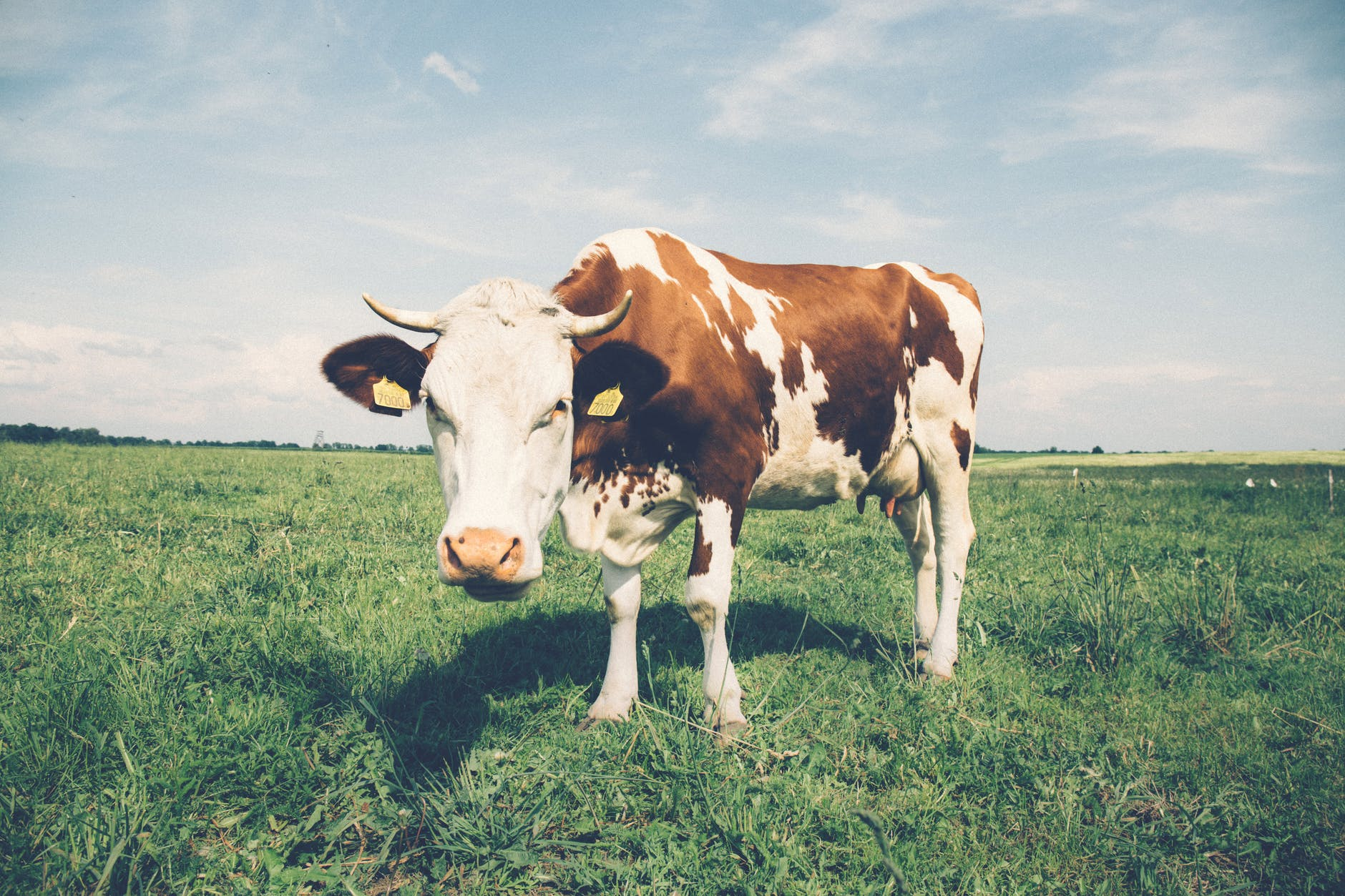# ScaleBasics
Scale refers to the ratio of image size to actual size.

To answer questions regarding scale, you will often need to work with ratio and proportion.

In order to cross-multiply, it is helpful to understand algebraic equations.  It is also helpful to know your multiplication and division tables.

Question
In the map above, the ratio of distance = 1 inch to 300 miles = 1:300 =$\bf\displaystyle\frac{1}{300}$ .
How many miles do 4 inches on the map represent?

1200 miles

To solve for an unknown variable in proportionate ratios, cross-multiply.$\bf\displaystyle\frac{a}{b}$$\bf\displaystyle\frac{c}{d}$
For ratios$\bf\displaystyle\frac{1}{300}$ and$\bf\displaystyle\frac{4}{d}$

bc = 300 × 4
1 × d = 300 × 4
1d = 1200
d = 1200

Question

In the map above, the ratio of distance = 1 inch to 300 miles = 1:300 =$\bf\displaystyle\frac{1}{300}$ .
Also in the map, Jackson, Mississippi and Columbia, South Carolina are approximately 2 inches apart.  In the real world, approximately how many miles are Jackson and Columbia apart?

600 miles

To solve for an unknown variable in proportionate ratios, cross-multiply.$\bf\displaystyle\frac{a}{b}$$\bf\displaystyle\frac{c}{d}$
For ratios$\bf\displaystyle\frac{1}{300}$ and$\bf\displaystyle\frac{2}{d}$

bc = 300 × 2
1 × d = 300 × 2
1d = 600
d = 600

Practice – Questions
1.  In the map above, the ratio of distance = 1 inch to 300 miles = 1:300 =$\bf\displaystyle\frac{1}{300}$ .
How many miles do 6 inches on the map represent?

2.  In the map above, the ratio of distance = 1 inch to 300 miles = 1:300 =$\bf\displaystyle\frac{1}{300}$ .
Also in the map, Columbus, Ohio and Tallahassee, Florida are approximately 3 inches apart.  In the real world, approximately how many miles are Columbus and Tallahassee apart?

3.  In the map above, the ratio of distance = 1 inch to 300 miles = 1:300 =$\bf\displaystyle\frac{1}{300}$ .
Also in the map, Montgomery, Alabama and Montpelier, Vermont are approximately 5 inches apart.  In the real world, approximately how many miles are Montgomery and Montpelier apart?

4.  In the image below, a cow is 1 inch long.  In the real world, a cow is 60 inches long.  Using a colon, write a scale representing this cow.5.  In the image below, a cow is 1 inch long.  In the real world, a cow is 60 inches long.  Using a fraction, write a scale representing this cow.5.$\bf\displaystyle\frac{1}{60}$